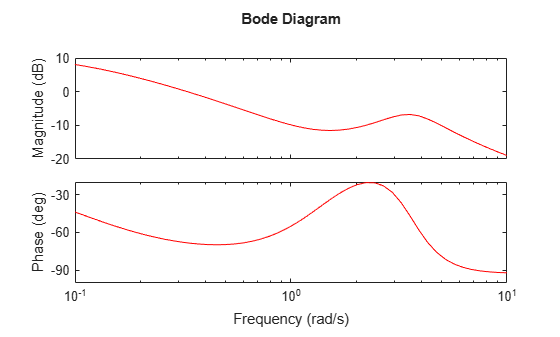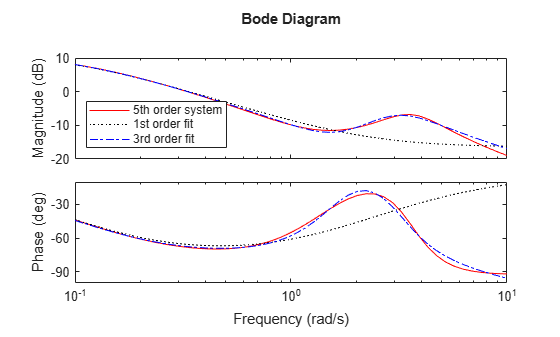# fitfrd

Fit frequency response data with state-space model

## Syntax

```B = fitfrd(A,N)
B = fitfrd(A,N,RD)
B = fitfrd(A,N,RD,WT)
```

## Description

`B = fitfrd(A,N)` is a state-space object with state dimension N, where `A` is an `frd` object and N is a nonnegative integer. The frequency response of `B` closely matches the D-scale frequency response data in `A`.

`A` must have either 1 row or 1 column, although it need not be 1-by-1. `B` will be the same size as `A`. In all cases, `N` should be a nonnegative scalar.

`B = fitfrd(A,N,RD)` forces the relative degree of `B` to be `RD`. `RD` must be a nonnegative integer. The default value for `RD` is 0. If `A` is a row (or column) then `RD` can be a vector of the same size as well, specifying the relative degree of each entry of `B`. If `RD` is a scalar, then it specifies the relative degree for all entries of `B`. You can specify the default value for `RD` by setting `RD` to an empty matrix.

`B = fitfrd(A,N,RD,WT)` uses the magnitude of `WT` to weight the optimization fit criteria. `WT` can be a `double, ss` or` frd`. If `WT` is a scalar, then it is used to weight all entries of the error criteria (`A-B`). If `WT` is a vector, it must be the same size as `A`, and each individual entry of `WT` acts as a weighting function on the corresponding entry of (`A-B`).

## Examples

collapse all

Use the `fitfrd` command to fit D-scale data.

Create D-scale frequency response data from a fifth-order system.

```sys = tf([1 2 2],[1 2.5 1.5])*tf(1,[1 0.1]); sys = sys*tf([1 3.75 3.5],[1 2.5 13]); omeg = logspace(-1,1); sysg = frd(sys,omeg); bode(sysg,'r-');```You can try to fit the frequency response D-scale data `sysg` with a first-order system, `b1`. Similarly, you can fit the D-scale data with a third-order system, `b3`.

```b1 = fitfrd(sysg,1); b3 = fitfrd(sysg,3);```

Compare the original D-scale data `sysg` with the frequency responses of the first and third-order models calculated by `fitfrd`.

```b1g = frd(b1,omeg); b3g = frd(b3,omeg); bode(sysg,'r-',b1g,'k:',b3g,'b-.') legend('5th order system','1st order fit','3rd order fit','Location','Southwest')```## Limitations

Numerical conditioning problems arise if the state order of the fit `N` is selected to be higher than required by the dynamics of `A`.

## Version History

Introduced before R2006a